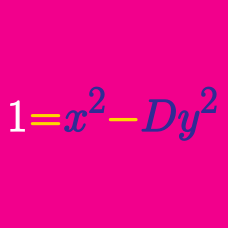Number Theory

# Quadratic Diophantine Equations: Level 4 Challenges

$4$ has the property that if one adds it to double its square, it yields a perfect square. In other words for natural numbers $m,n$:

$n^2 + n^2 + n = m^2$

There are four such $n<10^6$. If $4$ is the smallest $n$, what is the second smallest $n$?

What is the largest integer $\displaystyle n$ for which $n^2+24n+16$ is a perfect square?

$365$ can be written as a sum of $2$ consecutive perfect squares and also $3$ consecutive non-zero perfect squares: $365=14^2+13^2=12^2+11^2+10^2$

What is the next number with this property?

For how many positive integers $n<10^6$ is $2\times n! \times (n+2)!$ a perfect square?

Find the sum of all positive integers $m$ such that $2^m$ can be expressed as sums of four factorials (of positive integers).

Details and assumptions

The number $n!$, read as n factorial, is equal to the product of all positive integers less than or equal to $n$. For example, $7! = 7 \times 6 \times 5 \times 4 \times 3 \times 2 \times 1$.

The factorials do not have to be distinct. For example, $2^4=16$ counts, because it equals $3!+3!+2!+2!$.

×

Problem Loading...

Note Loading...

Set Loading...